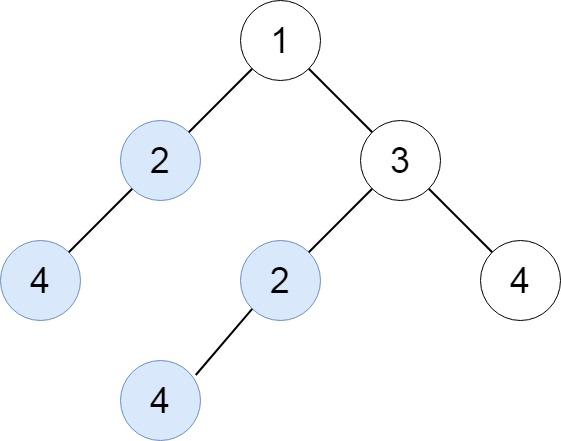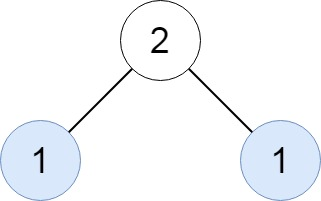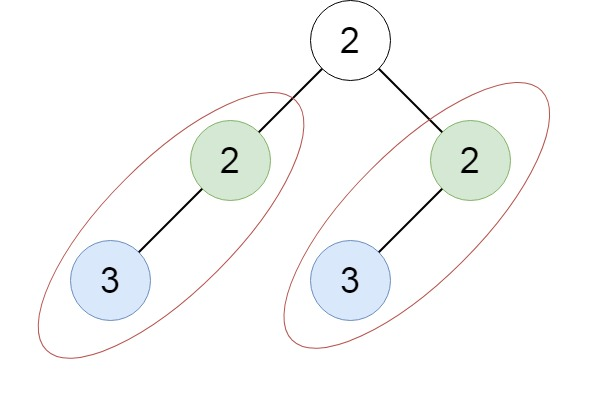GeetCode Hub

Given the root of a binary tree, return all duplicate subtrees.

For each kind of duplicate subtrees, you only need to return the root node of any one of them.

Two trees are duplicate if they have the same structure with the same node values.

Example 1:Input: root = [1,2,3,4,null,2,4,null,null,4]
Output: [[2,4],]

Example 2:Input: root = [2,1,1]
Output: []

Example 3:Input: root = [2,2,2,3,null,3,null]
Output: [[2,3],]

Constraints:

• The number of the nodes in the tree will be in the range [1, 10^4]
• -200 <= Node.val <= 200

/** * Definition for a binary tree node. * public class TreeNode { * int val; * TreeNode left; * TreeNode right; * TreeNode() {} * TreeNode(int val) { this.val = val; } * TreeNode(int val, TreeNode left, TreeNode right) { * this.val = val; * this.left = left; * this.right = right; * } * } */ class Solution { public List<TreeNode> findDuplicateSubtrees(TreeNode root) { } }